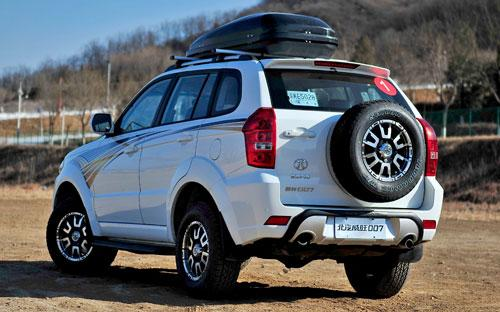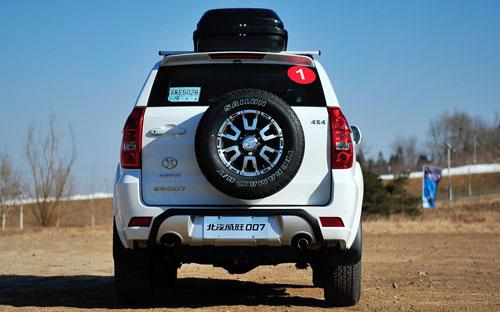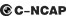## 北汽威旺 北汽威旺0070 种颜色可选2015款最低售价：10.98 万元起

4595(mm)1828(mm)1830(mm)##### 配置亮点：
• 胎压监测装置

• ISOFIX儿童座椅接口

• 车身稳定控制(ESC/ESP/DSC等)

• 电动天窗

• 定速巡航

• 后倒车雷达

• 真皮座椅

• GPS导航系统

• 氙气大灯

• 后视镜加热

2015款 2.0T 四驱豪华型 (55张)北汽威旺 北汽威旺007 在售车型

排量 车型 厂商指导价 本地最低报价 购车工具
2.0L 2.0L 两驱舒适型 5挡手动 8.98万 8.98万

询底价+对比
2.0T 2.0T 两驱豪华型 6挡手动 9.78万 9.78万

询底价+对比
2.0T 四驱豪华型 6挡手动 10.98万 10.98万

询底价+对比

北汽威旺 北汽威旺007 经销商

查看更多 >>
更多 >>

本地降价排名

### 北汽威旺 北汽威旺007 动力加速

北汽威旺007 0-100公里加速时间分布在 0.0-秒 属于 超跑级

动力级别 加速时间 车型

北汽威旺 北汽威旺007 视频

北汽威旺 北汽威旺007 新闻资讯

# “爸”气十足 威旺007车主谈“父亲节”购车心得

新闻中心 超过8633次关注

每年6月的第三个星期日，是父亲们的专属节日——父亲节。作为家里的顶梁柱，父亲总是表现出阳刚硬派的一面，一款“爸”气十足的SUV搭配父亲的身份堪称完美。凭借全...

# 北汽威旺007郑州上市

新闻中心 超过6860次关注

在5月20日这个甜蜜的日子里，“北汽威旺007河南上市发布会”在北汽威旺郑州顺之成汽车4S店成功举办，宣告北汽威旺旗下首款“8万元级全路况”SUV车型——威旺007面向...

# SUV市场“闯入者” 威旺007挑战哈弗H5

新闻中心 超过6708次关注

近日，随着北汽威旺全路况SUV威旺007的全面来袭，SUV市场沉浸在一片硝烟弥漫之中。北汽威旺007作为SUV市场的新晋闯入者，无论是外观设计、越野性还是8万元级的价格...

新闻中心 超过8719次关注

据悉，5月8日，北汽威旺旗下首款“8万元级全路况”SUV——北汽威旺007已于天猫商城首发上市。此次北汽威旺007共推出2.0L两驱手动舒适型、2.0T两驱手动豪华型及2.0T...

# 幸福如“7”而至 北汽威旺007邀你塞班双人游

新闻中心 超过6965次关注

继5月8日天猫首发后，北汽威旺007在全国各大区域火热上市，掀起抢购狂潮。现在，这股狂潮伴随着塞班岛的海风再次席卷而来，北汽威旺于6月1日至7月26日推出“北汽威...

# 北汽威旺007 VS长城哈弗H5

新闻中心 超过7041次关注

《速度与激情7》中的硬汉对决戏码刚刚谢幕，《复仇者联盟2》又吹响各路英雄聚首的集结号，在掀起新一轮观影高潮的同时，也刷新着中国电影票房的纪录。正是这份英雄...

# 售8.98-10.98万元 北汽威旺007正式上市

国产新车 超过67780次关注

日前，我们从天猫北汽威旺官方旗舰店获悉，北汽威旺007车型正式上市，新车搭载2.0L以及2.0T发动机，共包含有三款车型，售价区间为8.98-10.98万元。

猜你喜欢

﻿
• 快速找车
• 选择品牌
• 选择品牌
• A  奥迪
• A  阿斯顿·马丁
• A  阿尔法·罗密欧
• B  宝沃
• B  布加迪
• B  巴博斯
• B  保时捷
• B  宾利
• B  奔驰
• B  宝马
• B  本田
• B  别克
• B  标致
• B  比亚迪
• B  宝骏
• B  北汽制造
• B  北汽新能源
• B  北汽幻速
• B  北汽威旺
• B  北京汽车
• B  奔腾
• B  北汽绅宝
• C  长安
• C  长安商用
• C  长城
• C  昌河
• D  大众
• D  道奇
• D  DS
• D  东南
• D  东风风神
• D  东风风行
• D  东风小康
• D  东风风度
• D  东风
• F  福特
• F  丰田
• F  菲亚特
• F  法拉利
• F  福田
• F  福迪
• F  福汽启腾
• G  观致
• G  广汽传祺
• G  广汽吉奥
• G  GMC
• H  红旗
• H  汉腾汽车
• H  哈弗
• H  哈飞
• H  海格
• H  海马
• H  华颂
• H  黄海
• H  华泰
• H  恒天
• J  吉利汽车
• J  捷豹
• J  Jeep
• J  江淮
• J  江铃
• J  金杯
• J  九龙
• J  金旅
• K  凯翼
• K  凯迪拉克
• K  克莱斯勒
• K  科尼塞克
• K  卡威
• K  开瑞
• L  路虎
• L  林肯
• L  劳斯莱斯
• L  兰博基尼
• L  雷克萨斯
• L  铃木
• L  雷诺
• L  理念
• L  力帆
• L  莲花汽车
• L  猎豹
• L  路特斯
• L  陆风
• M  马自达
• M  MG
• M  MINI
• M  玛莎拉蒂
• M  摩根
• M  迈凯轮
• N  纳智捷
• O  欧宝
• O  讴歌
• O  欧朗
• Q  奇瑞
• Q  起亚
• Q  启辰
• R  日产
• R  荣威
• R  瑞麒
• S  三菱
• S  斯威汽车
• S  萨博
• S  smart
• S  斯柯达
• S  斯巴鲁
• S  思铭
• S  双龙
• S  上汽大通
• S  双环
• T  特斯拉
• T  腾势
• W  沃尔沃
• W  五菱汽车
• W  五十铃
• W  威兹曼
• W  威麟
• X  现代
• X  雪佛兰
• X  雪铁龙
• X  西雅特
• Y  一汽
• Y  英菲尼迪
• Y  英致
• Y  依维柯
• Y  野马汽车
• Y  永源
• Z  众泰
• Z  中华
• Z  中兴
• Z  知豆
• 选择车系
• 选择车系
• 车型对比
• 选择品牌
• 选择品牌
• A  奥迪
• A  阿斯顿·马丁
• A  阿尔法·罗密欧
• B  宝沃
• B  布加迪
• B  巴博斯
• B  保时捷
• B  宾利
• B  奔驰
• B  宝马
• B  本田
• B  别克
• B  标致
• B  比亚迪
• B  宝骏
• B  北汽制造
• B  北汽新能源
• B  北汽幻速
• B  北汽威旺
• B  北京汽车
• B  奔腾
• B  北汽绅宝
• C  长安
• C  长安商用
• C  长城
• C  昌河
• D  大众
• D  道奇
• D  DS
• D  东南
• D  东风风神
• D  东风风行
• D  东风小康
• D  东风风度
• D  东风
• F  福特
• F  丰田
• F  菲亚特
• F  法拉利
• F  福田
• F  福迪
• F  福汽启腾
• G  观致
• G  广汽传祺
• G  广汽吉奥
• G  GMC
• H  红旗
• H  汉腾汽车
• H  哈弗
• H  哈飞
• H  海格
• H  海马
• H  华颂
• H  黄海
• H  华泰
• H  恒天
• J  吉利汽车
• J  捷豹
• J  Jeep
• J  江淮
• J  江铃
• J  金杯
• J  九龙
• J  金旅
• K  凯翼
• K  凯迪拉克
• K  克莱斯勒
• K  科尼塞克
• K  卡威
• K  开瑞
• L  路虎
• L  林肯
• L  劳斯莱斯
• L  兰博基尼
• L  雷克萨斯
• L  铃木
• L  雷诺
• L  理念
• L  力帆
• L  莲花汽车
• L  猎豹
• L  路特斯
• L  陆风
• M  马自达
• M  MG
• M  MINI
• M  玛莎拉蒂
• M  摩根
• M  迈凯轮
• N  纳智捷
• O  欧宝
• O  讴歌
• O  欧朗
• Q  奇瑞
• Q  起亚
• Q  启辰
• R  日产
• R  荣威
• R  瑞麒
• S  三菱
• S  斯威汽车
• S  萨博
• S  smart
• S  斯柯达
• S  斯巴鲁
• S  思铭
• S  双龙
• S  上汽大通
• S  双环
• T  特斯拉
• T  腾势
• W  沃尔沃
• W  五菱汽车
• W  五十铃
• W  威兹曼
• W  威麟
• X  现代
• X  雪佛兰
• X  雪铁龙
• X  西雅特
• Y  一汽
• Y  英菲尼迪
• Y  英致
• Y  依维柯
• Y  野马汽车
• Y  永源
• Z  众泰
• Z  中华
• Z  中兴
• Z  知豆
• 选择车系
• 选择车系
• 选择车型
• 选择车型
• 意见反馈